Question

# A variable of a population has a mean of 100 and a standard deviation of 28....

1. A variable of a population has a mean of 100 and a standard deviation of 28. Consider the sample distribution of samples means of sample size 49.

a. What is the mean of sample means?
b. What is the standard deviation of the sample means?
c. Is the distribution of sample means normal? Why or why not? Do we need to know if the variable in question is normally distributed in the population? Why or why not?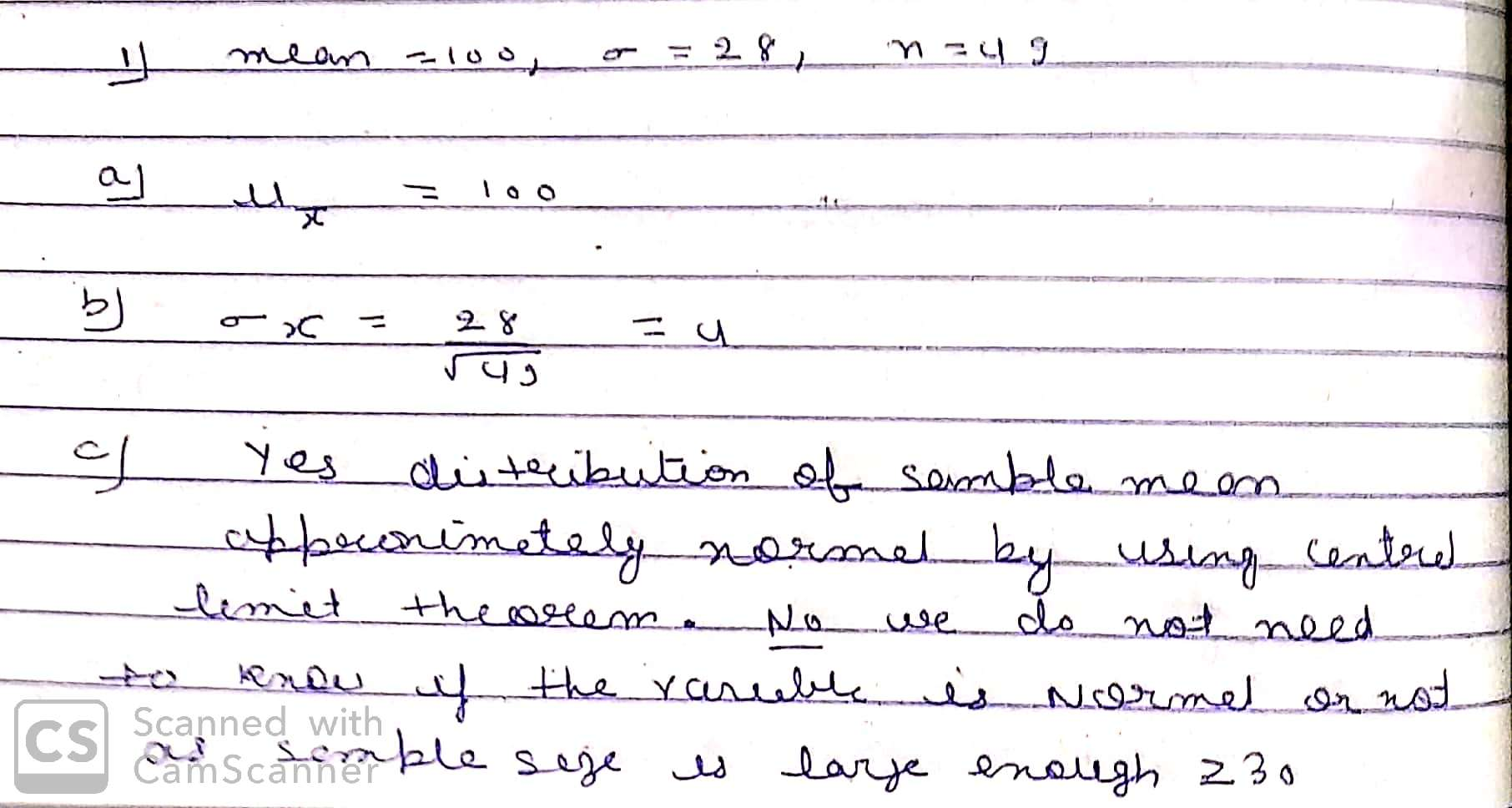#### Earn Coins

Coins can be redeemed for fabulous gifts.

Similar Homework Help Questions
• ### A variable of a population has a mean of μ=100 and a standard deviation of σ=35....

A variable of a population has a mean of μ=100 and a standard deviation of σ=35. a. The sampling distribution of the sample mean for samples of size 49 is approximately normally distributed with mean________ and standard deviation_______?

• ### A variable of a population has a mean of u = 70 and a standard deviation...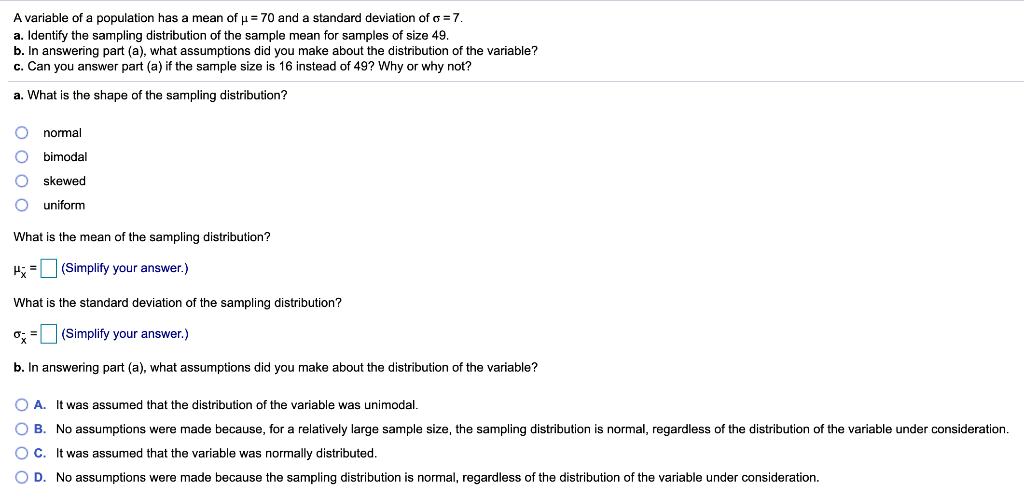A variable of a population has a mean of u = 70 and a standard deviation of a = 7. a. Identify the sampling distribution of the sample mean for samples of size 49. b. In answering part (a), what assumptions did you make about the distribution of the variable? c. Can you answer part (a) if the sample size is 16 instead of 49? Why or why not? a. What is the shape of the sampling distribution? normal a...

• ### a variable of a population has a mean of 79 and a standard deviation of 18....

a variable of a population has a mean of 79 and a standard deviation of 18. a. Identify the sampling distribution of the sample mean for samples of size 81. b. In answering part​ (a), what assumptions did you make about the distribution of the​ variable? c. Can you answer part​ (a) if the sample size is 25 instead of ​81? Why or why​ not? a. What is the shape of the sampling​ distribution? uniform bimodal skewed normal? What is...

• ### 5.4.1 Question Help A population has a mean = 141 and a standard deviation o =...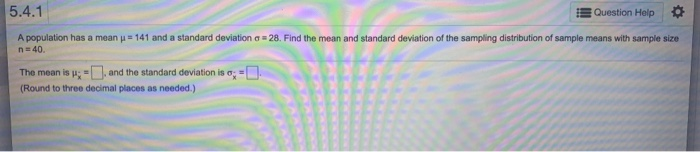5.4.1 Question Help A population has a mean = 141 and a standard deviation o = 28. Find the mean and standard deviation of the sampling distribution of sample means with sample size n = 40. The mean is :-), and the standard deviation is 0;=0 (Round to three decimal places as needed.) 5.4.2 Question Help A population has a meanu - 74 and a standard deviation = 8. Find the mean and standard deviation of a sampling distribution of...

• ### If the standard deviation of a normally distributed population is 60 and we take a sample...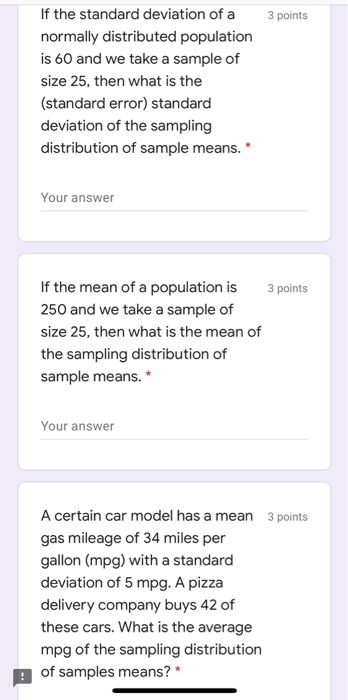If the standard deviation of a normally distributed population is 60 and we take a sample of size 25, then what is the (standard error) standard deviation of the sampling distribution of sample means. * Your answer If the mean of a population is 3 points 250 and we take a sample of size 25, then what is the mean of the sampling distribution of sample means. * Your answer 3 points A certain car model has a mean gas...

• ### Consider a population with a quantitative variable for which we would like to estimate ne population...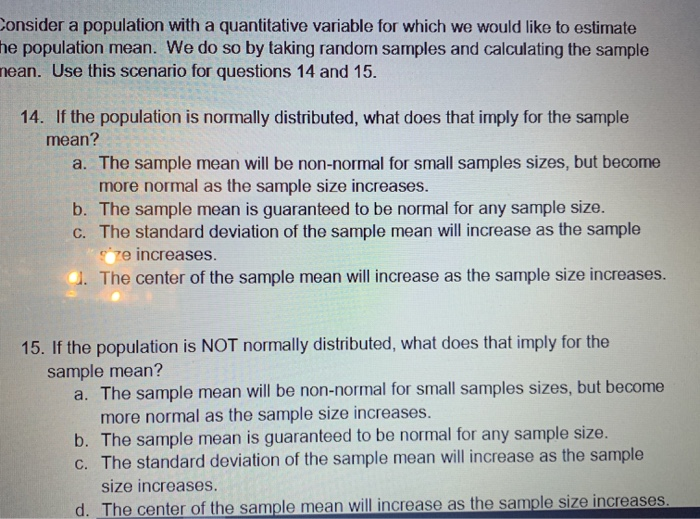Consider a population with a quantitative variable for which we would like to estimate ne population mean. We do so by taking random samples and calculating the sample nean. Use this scenario for questions 14 and 15. 14. If the population is normally distributed, what does that imply for the sample mean? a. The sample mean will be non-normal for small samples sizes, but become more normal as the sample size increases. b. The sample mean is guaranteed to be...

• ### A population is normally distributed with a mean of 100 and a standard deviation of 10, for sampl...

A population is normally distributed with a mean of 100 and a standard deviation of 10, for samples of size 25, what is the probability of randomly sampling and getting a sample mean of 103 or more?

• ### Let z be a normal random variable with mean 0 and standard deviation 1. What is...

Let z be a normal random variable with mean 0 and standard deviation 1. What is P(z > -1.1)? -0.8643 0.8643 -0.1357 0.1357 If all possible random samples of size n are taken from a population that is not normally distributed, and the mean of each sample is determined, what can you say about the sampling distribution of sample means? It is approximately normal provided that n is large enough. It is positively skewed. It is negatively skewed. None of...

• ### A population has a mean μ-85 and a standard deviation σ-21. Find the mean and standard...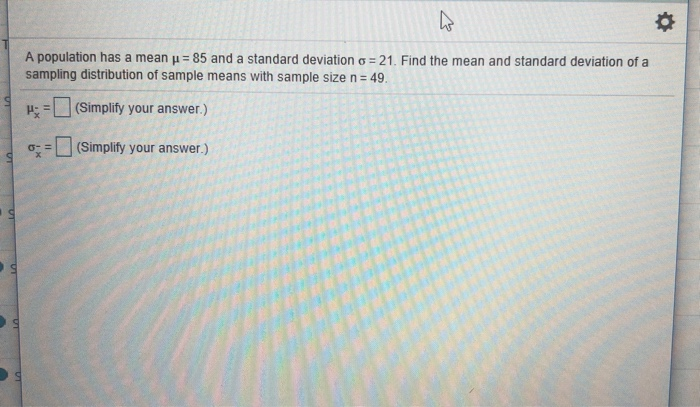A population has a mean μ-85 and a standard deviation σ-21. Find the mean and standard deviation of a sampling distribution of sample means with sample size n 49 H(Simplity your answer) o-# L] (Simplify your answer.) to the random variable x is normally distributed with mean-83 and standard deviation ơ-4 Find the indicated probability P(70sx 76) P(70 < x < 76)= Round to four decimal places as needed.) Enter your answer in the answer box

• ### Question 10 14 pts Suppose X is a random variable with mean 100 and standard deviation...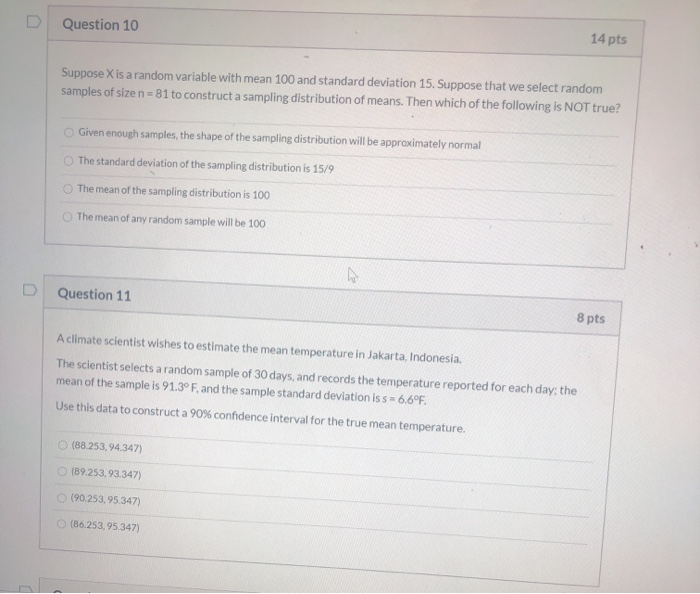Question 10 14 pts Suppose X is a random variable with mean 100 and standard deviation 15. Suppose that we select random samples of size n=81 to construct a sampling distribution of means. Then which of the following is NOT true? Given enough samples, the shape of the sampling distribution will be approximately normal The standard deviation of the sampling distribution is 15/9 The mean of the sampling distribution is 100 The mean of any random sample will be 100...